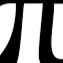# Unresolved Detail In Plotted Equations

Sometimes the calculator detects that an equation is too complicated to plot perfectly in a reasonable amount of time. When this happens, the equation is plotted at lower resolution.

Was this article helpful?
221 out of 246 found this helpful
Have more questions? Submit a request

#### 927 Comments

•Owen J
•Ekremer

\frac{\left(\cos x^3\right)}{\left(\sin y^2\right)}+3y\sin x=\frac{\sin y}{x!}

What the heck even is this

•nyerka nyerka

CONFETTI!!!!!!!!!!
\sum_{n=1}^0n!=\frac{\left(xy\frac{\left(\cos x^3\right)}{\left(\sin y^2\right)}\cdot\frac{1}{\sin\left(y\right)}\right)}{2\tan x^9y^8}

•nyerka nyerka

This one took around 20 minutes.

https://www.desmos.com/calculator/cwrsdfjl9v

•Blue DragonFire
•Ekremer

ARE YOU READY FOR MADNESS?????
\tan\left(\frac{\frac{\pi\cos x}{y}}{b}\right)=\sin^{-1}\left(\frac{\frac{\frac{\cos y}{\pi}\tan\left(x\right)}{x\pi\left(\frac{\tan x}{2\pi y\ }\right)}}{a}\right)

•Tanksear Industries

This. (Built on yours, Ekremer).

https://www.desmos.com/calculator/8qgs9gemuf

•mATT C
•Tanksear Industries
•Tanksear Industries

Look at the orange part. Then look at the black part. Then realize that this is just a bunch of rectangles.

https://www.desmos.com/calculator/onux9vdhvi

•Tanksear Industries

This will bring joy to both your processor and your graphics card.

https://www.desmos.com/calculator/pyaje7r5yn

•Tanksear Industries

Is this my forum now?

•Lio Oms

y=xyxyxyxyxyyyxyxyxyxyxyxxy^{xyxyyyyyx}

y=69x\cdot x^{420}-\frac{24x}{4xyxyxyx\cdot69xxy}\cdot x^{69^{x^{yy}}}

y=69x\cdot420x^{69}+\frac{\frac{666}{xxyyxxyyx}}{xxxxx+420860xy}

y=x^{165413047\cdot41234xx}yy+xxxx-\frac{yxxx}{420000}

oof

•mATT C

sin \left(\frac{\left(x-10\right)y}{10}\right)=\frac{-\sin\left(x+8\right)}{\cos\left(2x+20\right)}

Edited by mATT C
•J S (bin4rym4ge)

0.5=\left(\cos \left(x\right)+\cos \left(y\sin \left(\frac{\pi }{5}\right)+x\cos \left(\frac{\pi }{5}\right)\right)+\cos \left(y\sin \left(\frac{2\pi }{5}\right)+x\cos \left(\frac{2\pi }{5}\right)\right)+\cos \left(y\sin \left(\frac{3\pi }{5}\right)+x\cos \left(\frac{3\pi }{5}\right)\right)+\cos \left(y\sin \left(\frac{4\pi }{5}\right)+x\cos \left(\frac{4\pi }{5}\right)\right)\right)

•Joey Hughes

https://www.desmos.com/calculator/3na7yomuvg is a very adjustable fine detail graph that isn't just a garbled mess.

•mATT C

Weird Landscaping:

https://www.desmos.com/calculator/j8pix4cchm•Kerndr

y/x=log(y*sin(x))*lxyl*cos(y) is all I have to say.

•Ze_Mathster

Today we are bringing back the idea. Have fun folks!

cos(xy)sec^-1(xcoth(x^y))=sin(y^x)

It killed desmos.Mmmm?

•Ze_Mathster
Edited by Ze_Mathster
•22gverrillgrant

sin(c*y)=cos(x*j) and turn the sliders on.

•GlitchedGraph666

\cos\left(x+y-x^2+\sin\left(x\right)\right)=\cos\left(y\right)

•GlitchedGraph666

x\cdot\sin\left(y!\right)+\cos\left(x\cdot y\right)=\sin\left(xy\right)

\sqrt{\sin\left(x\right)\cos\left(y\right)\cdot9}<2

\frac{7}{x}+\sin\left(y\right)\cdot x+\cos\left(xy\right)=\tan\left(xy\right)

x\cdot-\sin\left(x\right)\cdot\sin\left(2\cdot y\right)=\sin\left(xy\right)

x^2+\sin\left(x\cdot y\right)-x+\cos\left(x\right)=\cos\left(x!\right)\cdot xy^2

•8020727

\frac{\frac{x}{2}}{\tan\left(\frac{\left(\frac{360}{y}\right)}{2}\right)}\cdot x\cdot\frac{1}{2}\cdot y\cdot2x\cdot\frac{1}{3}=192\sqrt{3}

•Tanksear Industries

https://www.desmos.com/calculator/pudkdyz4ia

Anyone who's played BotW will know what this looks like...

•Tanksear Industries

\frac{\tan}{\frac{888xa}{\frac{\left|a\cdot\sin\left(x\right)\right|}{\operatorname{floor}\left(\sec\left(x\right)\right)}}}

This is a bit of a tangled mess that renders semi-properly for about 5 seconds, then turns into some sort of... thing.

*Update*

This crashes Desmos.

Edited by Tanksear Industries
•Tanksear Industries

y=\tan(x^2+2^y)

•Tanksear Industries

https://www.desmos.com/calculator/5duhmnlt20

Not that bad, as surrealism goes...

(Yes, I've had a dream like this before)

•GlitchedGraph666

x\cdot\sin\left(y\right)=y\cdot\sin\left(x\right)

Quite 3D looking.

•Jwitte038

\frac{\sin y}{\tan x}=\frac{x}{\tan y}

Article is closed for comments.